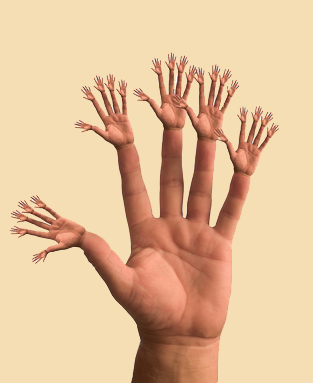﻿

# The (hexa)decimal system

## The decimal system

• Etymology
• decimal:
c.1600, from M.L. decimalis "of tithes or tenths,"
• digital:
1650s, "pertaining to fingers," from L. digitalis, from digitus (see digit).

The decimal system is called base 10. There are 10 digits (0-9) in base 10.

0 1 2 3 4 5 6 7 8 9

We've probably adopted the decimal system because we have 10 fingers.If we had thirty fingers on each hand instead of five, we would probably use the Babylonian sexagesimal system (base 60).

Beyond the fact that we have ten fingers, the distinction between 1 and 0 is remarkably convenient. Count the zeroes:

• 101 * 101 = 102 . . . 100
• 103 * 104 = 107 . . . 10,000,000

To describe a group of 125 objects in base 10 requires 3 digits:

• 1 * 102 = 100
• 2 * 101 = 20
• 5 * 100 = 5

The distinction between 1 and 0 has inspired philosophers for centuries.

 103 102 101 100 0 0 0 1 one 0 0 1 0 ten 0 1 0 0 (a/one) hundred 1 0 0 0 (a/one) thousand

After the numbers, the alphabet is used for successive digits. For base 16, we need six more symbols:

 Hexadecimal A B C D E F Decimal 10 11 12 13 14 15

In base 16, a group of 256 objects is described using 3 digits:

• 1 * 162 = 256
• 0 * 161 = 0
• 0 * 160 = 0

To describe a group of 255 objects in hexadecimal only requires two digits:

• 0 * 162 = 0
• F * 161 = 240
• F * 160 = 15

FF FF FF is the color code for pure white on the web. Learn more about the colors of the web on Roland Dassonval's well-made site (in French)

 163 162 161 160 base 10 0 0 0 1 1 0 0 1 0 16 0 1 0 0 256 1 0 0 0 4096